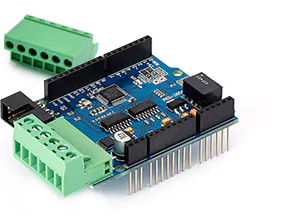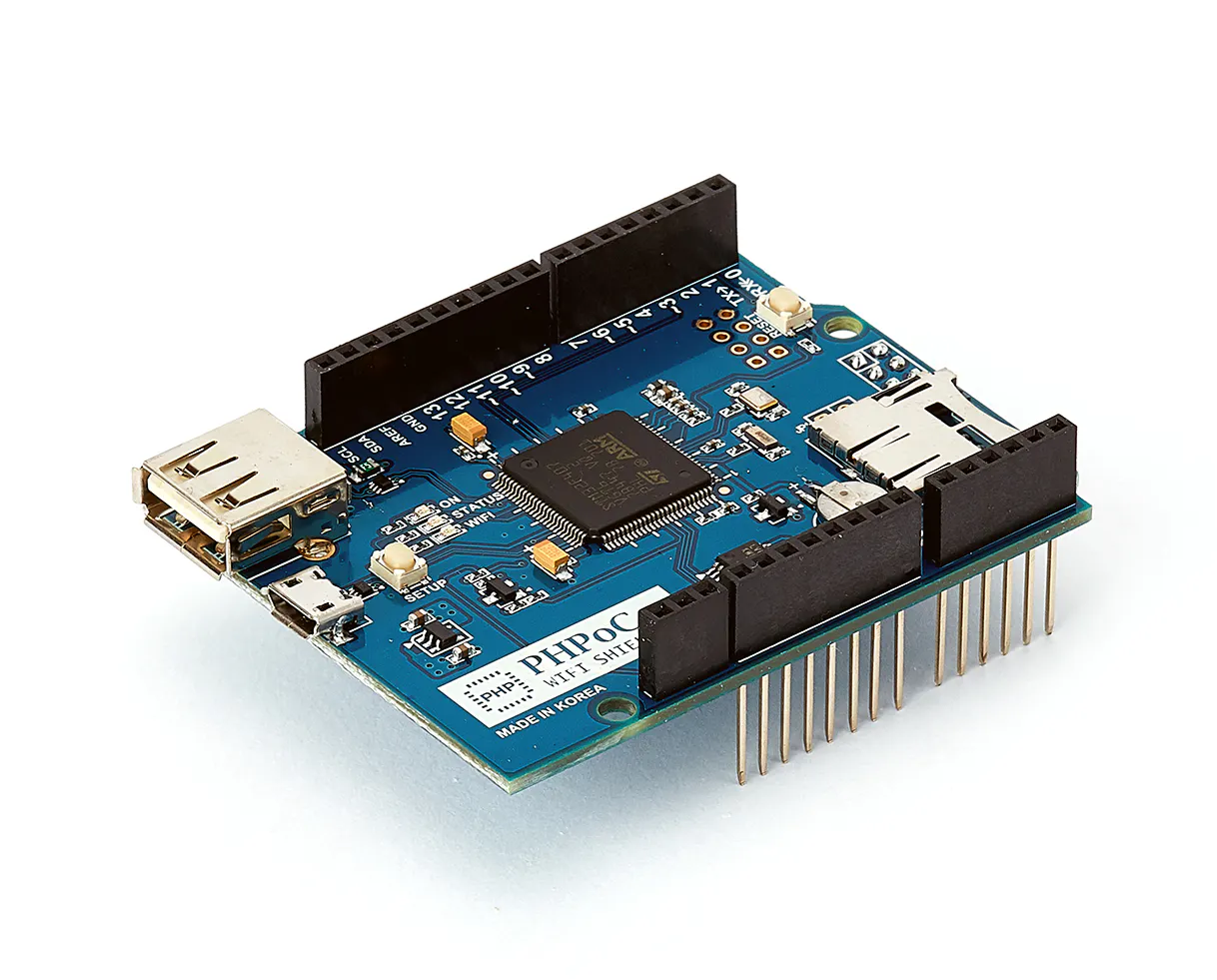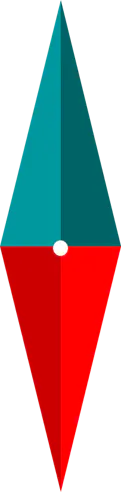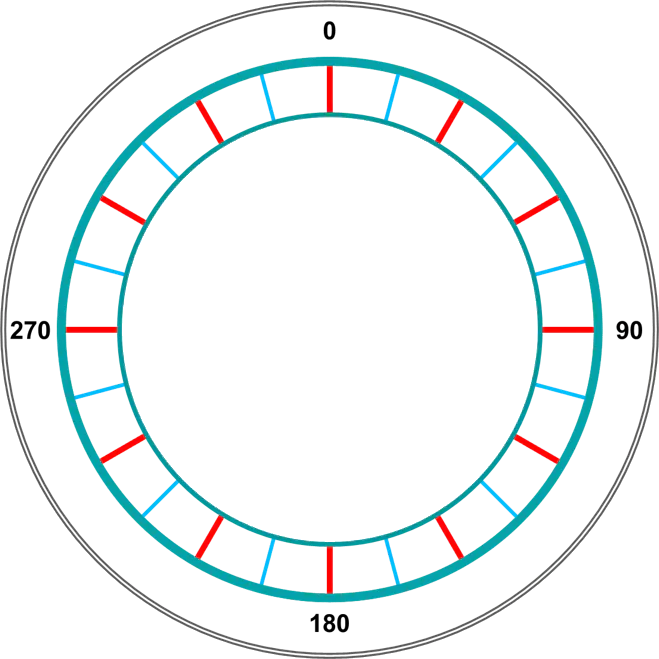# Arduino - Control Position of DC Motor Precisely via Web

Control DC motor using PID controller via web.

IntermediateFull instructions provided7,720

## Things used in this project

### Hardware components

 DC motor with encoder
×1PHPoC DC Motor Controller
×1PHPoC WiFi Shield 2 for Arduino
×1Arduino UNO & Genuino UNO
×1

## Schematics

### needle.png### plate.png## Code

### ArduinoCode

Arduino
This is arduino code, which run in infinite loop.
```#include <Phpoc.h>
#include <PhpocExpansion.h>
#include <Pid.h>

PhpocServer server(80);
DcMotorPID dcPid1(1, 1);
long targetPosition;

void setup(){
Serial.begin(9600);
while(!Serial)
;

//Phpoc.begin(PF_LOG_SPI | PF_LOG_NET);
Phpoc.begin();
server.beginWebSocket("remote_dc");

Serial.println(Phpoc.localIP());

Expansion.begin(460800);

dcPid1.setPeriod(10000);
dcPid1.setEncoderPSR(64);
dcPid1.setDecay(0);
dcPid1.setFilterFrequency(5000);
dcPid1.setScaleFactor(5); // depending on motor, should test manually to have the best value
dcPid1.setIntegralLimit(-2000, 2000);
dcPid1.begin(PID_POSITION);

/*----------- check enc pol-------------- */
int pwm = 0; long pos = 0;
while(!pos)
{
pwm += 10;
dcPid1.setWidth(pwm);
pos = dcPid1.getEncoderPosition();
delay(1);
}

if(pos < 0)
dcPid1.setEncoderPolarity(-1);

dcPid1.setWidth(0);

//dcPid1.setGain(24.099171193725, 10.78947680748, 0.0617886);

/* PID auto-tuning */
dcPid1.beginTune();
while(!dcPid1.loopTune())
;

dcPid1.setEncoderPosition(0);

Serial.print(F("PID TUNED Kp: "));Serial.println(dcPid1.getKp());
Serial.print(F("PID TUNED Ki: "));Serial.println(dcPid1.getKi());
Serial.print(F("PID TUNED Kd: "));Serial.println(dcPid1.getKd());
}

void loop(){
PhpocClient client = server.available();

if(client) {

if(data) {
float targetAngle = data.toFloat();
targetPosition = (long)(targetAngle / 360.0 * (13 * 100 * 4));
}
}

long position = dcPid1.loop(targetPosition);

//Serial.print(targetPosition);
//Serial.print(" ");
//Serial.println(position);
}
```

### remote_dc.php

PHP
remote_dc.php is a file that contains Web User Interface. It needs to be stored on PHPoC [WiFi] Shield. In order to upload the file to PHPoC [WiFi] Shield, please do the following steps:
- Copy the below code and save it into remote_dc.php file.
- Install PHPoC Debugger
- Connect PHPoC Debugger to PHPoC [WiFi] Shield via micro-USB cable according to this instruction: https://www.phpoc.com/support/manual/phpoc_debugger_manual/contents.php?id=ready
- Note that Arduino must be powered.
- Upload remote_dc.php file to PHPoC [WiFi] Shield according to this instruction: https://www.phpoc.com/support/manual/phpoc_debugger_manual/contents.php?id=major_upload
```<!DOCTYPE html>
<html>
<title>PHPoC / <?echo system("uname -i")?></title>
<meta name="viewport" content="width=device-width, initial-scale=0.7">
<style>
body { text-align: center; background-color: whiite;}
canvas {
background: url(plate.png) no-repeat;
background-size: contain;}
}
</style>
<script>
var CANVAS_WIDTH, CANVAS_HEIGHT;
var PIVOT_X, PIVOT_Y;
var PLATE_WIDTH = 659.0, PLATE_HEIGHT = 569.0; // size of background image
var NEEDLE_WIDTH = 121, NEEDLE_HEIGHT = 492; // size of needle image
var RATIO;
var plateAngle = 0;
var needleImage = new Image();
var clickState = 0;
var lastAngle = 0;
var mouse_xyra = {x:0, y:0, r:0.0, a:0.0};
var ws;

needleImage.src = "needle.png";

function init() {
CANVAS_WIDTH = window.innerWidth - 50;
CANVAS_HEIGHT = window.innerHeight - 50;

if(CANVAS_WIDTH < CANVAS_HEIGHT)
CANVAS_HEIGHT = CANVAS_WIDTH;
else
CANVAS_WIDTH = CANVAS_HEIGHT;

PIVOT_X = CANVAS_WIDTH/2;
PIVOT_Y = CANVAS_HEIGHT/2;

RATIO = CANVAS_WIDTH / PLATE_WIDTH;

NEEDLE_WIDTH = Math.round(NEEDLE_WIDTH * RATIO);
NEEDLE_HEIGHT = Math.round(NEEDLE_HEIGHT * RATIO);

var canvas = document.getElementById("canvas");

canvas.width = CANVAS_WIDTH;
canvas.height = CANVAS_HEIGHT;

var ctx = canvas.getContext("2d");

ctx.translate(PIVOT_X, PIVOT_Y);

rotate_plate(0);

ws = new WebSocket("ws://<?echo _SERVER("HTTP_HOST")?>/remote_dc", "text.phpoc");
document.getElementById("ws_state").innerHTML = "CONNECTING";

ws.onopen  = function(){ document.getElementById("ws_state").innerHTML = "OPEN" };
ws.onclose = function(){ document.getElementById("ws_state").innerHTML = "CLOSED"};
ws.onerror = function(){ alert("websocket error " + this.url) };

ws.onmessage = ws_onmessage;
}
function ws_onmessage(e_msg) {
e_msg = e_msg || window.event; // MessageEvent

plateAngle = Number(e_msg.data);
rotate_plate(plateAngle);
}
function rotate_plate(angle) {
var canvas = document.getElementById("canvas");
var ctx = canvas.getContext("2d");

ctx.clearRect(-PIVOT_X, -PIVOT_Y, CANVAS_WIDTH, CANVAS_HEIGHT);
ctx.rotate(-angle / 180 * Math.PI);
ctx.drawImage(needleImage, 0, 0, needleImage.width, needleImage.height, -NEEDLE_WIDTH/2, -NEEDLE_HEIGHT/2, NEEDLE_WIDTH, NEEDLE_HEIGHT);
ctx.rotate(angle / 180 * Math.PI);

debug = document.getElementById("debug");
debug.innerHTML = plateAngle.toFixed(1);
}
function check_update_xyra(event, mouse_xyra) {
var x, y, r, a;
var min_r, max_r, width;

if(event.touches) {
var touches = event.touches;

x = (touches.pageX - touches.target.offsetLeft) - PIVOT_X;
y = PIVOT_Y - (touches.pageY - touches.target.offsetTop);
}
else {
x = event.offsetX - PIVOT_X;
y = PIVOT_Y - event.offsetY;
}

/* cartesian to polar coordinate conversion */
r = Math.sqrt(x * x + y * y);
a = Math.atan2(y, x);

mouse_xyra.x = x;
mouse_xyra.y = y;
mouse_xyra.r = r;
mouse_xyra.a = a;

return true;
else
return false;
}
function mouse_down() {
if(event.touches && (event.touches.length > 1))
clickState = event.touches.length;

if(clickState > 1)
return;

if(check_update_xyra(event, mouse_xyra)) {
clickState = 1;
lastAngle = mouse_xyra.a / Math.PI * 180.0;
}
}
function mouse_up() {
clickState = 0;
}
function mouse_move() {
var angle, angleOffset;

if(event.touches && (event.touches.length > 1))
clickState = event.touches.length;

if(clickState != 1)
return;

if(!check_update_xyra(event, mouse_xyra)) {
clickState = 0;
} else {

angle = mouse_xyra.a / Math.PI * 180.0;

if(angle < 0.0)
angle = angle + 360.0;

angleOffset = angle - lastAngle;
lastAngle = angle;

if(angleOffset > 180.0)
angleOffset = -360.0 + angleOffset;
else
if(angleOffset < -180.0)
angleOffset = 360 + angleOffset;

plateAngle += angleOffset;
rotate_plate(plateAngle);

if(ws != null & ws.readyState == 1)
ws.send(plateAngle.toFixed(4) + "\r\n");
}
event.preventDefault();
}
</script>

<body>
<h2>

Arduino - DC Motor Position Control<br><br>
<canvas id="canvas"></canvas>
<p>
WebSocket : <span id="ws_state">null</span><br>
Angle : <span id="debug">0</span>
</p>
</h2>
</body>

</html>
```

## Credits

### IoT_lover

11 projects • 147 followers